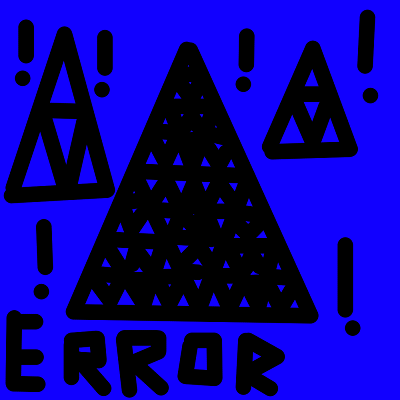## On the Subject of Inverse

This module makes complete sense... NOT!

This module contains a symbol at the top middle and buttons around the symbol. The goal is to press the correct button from the corresponding number from the symbol.

### Step 1: Symbol To Number

A symbol will either correspond to a number OR an error. If the symbol shows an error, skip to ‘Step ERROR’.

01234567891011Error 1Error 2### Step 2: Unmodifying with inverse

Take the number and use the bullet points below. However, the bullet points tell how the number was modified, so you must use invert the instructions to UNMODIFY the number. Remember that ‘the number’ is the widget count, NOT the display num. Find the first statement that applies.

• A widget will be chosen as the number. If the number is 0, 1, or 2, it is not modified.
• If the number is between 3 and 5 inclusive, the module has multiplied the number by 2 and added 1.
• If the number is between 6 and 8 inclusive, the module has took away 3 from the number.
• If the number is between 9 and 11 inclusive, the module has multiplied the number by 2 and took away 12 from the number.
• If the number is between 12 and 20 inclusive, the module has multiplied the number by 2 and took away 3 from the number. HOWEVER, this bullet point won't be much of importance.
• If the number is bigger than 20, the module has multiplied it by 3. HOWEVER, this bullet point won't be much of importance either.

### Step 3: Solving

Now that you have found the originating number, there will be widget/s that have this amount. However, the widget/s must be one of these: ports (TL button), port plates (TM button), batteries (TR button), battery holders (BL button), unlit indicators (BM button), or lit indicators (BR button). After that, just press the corresponding button.

If there are multiple corresponding buttons that should work, just press one of them.

### Step ERROR: Otherwise

If you have had to come to this step, this has meant that the display was showing an error.

If ERROR 1 was shown, press the otherwise button when the ones of seconds on the timer is equal to the amount of batteries modulo 10.

If ERROR 2 was shown, press the otherwise button when the ones of seconds on the timer is equal to the amount of strikes modulo 10.